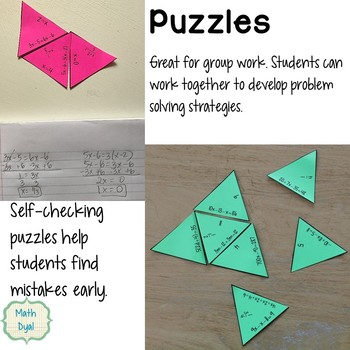# Solve Equations Activity Bundle

Rated 4.9 out of 5, based on 33 reviews
33 Ratings;
8th - 10th
Subjects
Resource Type
Formats Included
• Zip
\$16.00
List Price:
\$25.00
You Save:
\$9.00
Bundle
\$16.00
List Price:
\$25.00
You Save:
\$9.00
Bundle
Report this resource to TPT

#### What educators are saying

I used multiple resources out of this pack. I am glad that I made this purchase. I used it for one unit in my 8th grade class and I'm planning to use it with my 7th graders later in the year.

#### Products in this Bundle (11)

showing 1-5 of 11 products

### Description

Have your students practice solving equations these fun, self-checking activities that increase in complexity - four coloring activities for independent practice and three puzzles for group work! In each of the coloring activities: students answer each problem and then color that piece of the picture according to their coloring chart. In the puzzles, they solve problems and match equations pieces to solutions. Students can self-correct their work when their answer doesn't match one of the choices.

This bundle includes NINE activities:

-Solving Equations with Symbols Puzzle is a great way to introduce your students to solving equations with problems that can be solved using mental math.

-Solving Two-Step Equations Coloring Activity includes equations with negative numbers and solving using multiplication and division.

-Solving Equations with Variables on Both Sides Coloring Activity includes combining like terms and distributive property as well as no solution and all real number solutions.

-Solving Multi-Step Equations Coloring Activity includes problems with distributive property, variables on both sides, combining like terms, negative numbers, and all the properties of equality.

- Solving Equations with Division Puzzle features equations with three different types of division: when the variable is the numerator, when the variable is in an expression in the numerator, and fractional coefficients.

- Solving Equations Puzzle is a hands-on activity for students to practice solving equations using the distributive property and combining like terms. This activity promotes active engagement and mathematical discussions as students work together to match their problems and answers and solve the puzzle.

- Solve Equations Winter Coloring Activity allows students to practice solving equations with fractions, division, combining like terms, variables on both sides, distributive property and adding integers with this self-checking activity. Students solve each of the four equations in a box, then sum their answers. They then color the snowflake that matches their sum the color they choose.

-- Solve Multi-Step Equations Puzzle allows students to practice solving equations with fractions, division, combining like terms, variables on both sides, and distributive property by working together to assemble a puzzle.

* Solve Equations with Variables on Both Sides and Combining Like Terms allows students to practice solving equations using the procedures for variables on both sides vs combining like terms.

Total Pages